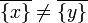# Kolmogorov space

(Redirected from T0 space)

## Definition

A topological space$X$ is termed a$T_0$ space or Kolmogorov space if it satisfies the following equivalent conditions:

1. For any two distinct points$x,y \in X$, there is either an open subset containing$x$ but not$y$, or an open subset containing$y$ but not$x$.
2. For any two distinct points$x,y \in X$, there is either a closed subset containing$x$ but not$y$, or a closed subset containing$y$ but not$x$.
3. For any two distinct points$x,y \in X$ such that$\overline{\{ x \} } \ne \overline{ \{ y \} }$, i.e., the closures of any two distinct one-point subsets must be distinct.
This article defines a property of topological spaces: a property that can be evaluated to true/false for any topological space|View a complete list of properties of topological spaces

In the T family (properties of topological spaces related to separation axioms), this is called: T0

## Relation with other properties

### Stronger properties

Property Meaning Proof of implication Proof of strictness (reverse implication failure) Intermediate notions
T1 space singleton subsets are closed Kolmogorov not implies T1
Hausdorff space KC-space, Locally Hausdorff space, Sober T0 space|FULL LIST, MORE INFO